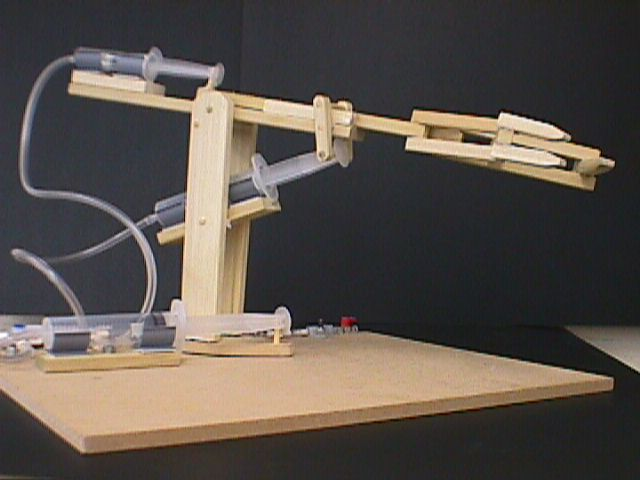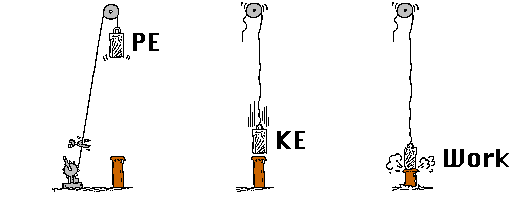Efficiency...Efficiency...Efficiency
?
?
?
?
?WHAT SHOULD YOU KNOW:
You should know that efficiency is a comparison of the useful work energy provided by a machine or system to the work energy applied to the machine or system.

You should know that the formula for efficiency is:

You should know that the parts of a machine or system and how they are connected together will affect the machine's or system's efficiency.

You should know that other forces such as friction will affect an object's movement.

You should know that velocity ratio is a comparison of the distance a load moves to the distance travelled by the force needed to move it.
Energy output
Energy input
X  100%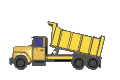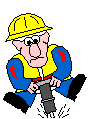POWER
tells us how fast work is being done.
To calculate power, divide the work done by the time it takes to do it. ( P = Work / Time )
The unit for power is the watt (w). The unit for work is the joule (J). Time is measured in seconds (sec). That means that one watt is equaled to one joule per second.
Power (W) = Work (J) / Time (sec)
WORK
and the measure of the distance the object moves. Work = force X distance.  Force is measured in Newtons, distance is measured in metres and one Newton metre is equal to one joule. Joule is the amount of work done BUT if work is to be done, then the object has to be moved. If you spent 1/2 hour pushing on a parked car, and you were tired and hot and sweaty, you may feel like you have worked but if the car never moved then you did NO work.
is the result of a force applied to the object
See that dump truck at the top of the page, or that little man playing with the jack-hammer - how do you think those machines work??
HINT!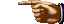The dump-truck is hydraulics. The jack-hammer is pneumatics. It is time to design a device that uses a mechanical system which is operated by one of the above.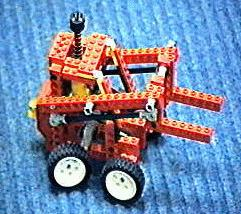Mechanical Energy in the form of Potential Energy
Force applied, it does Work
Mechanical Energy in the form of Kinetic Energy
What are the costs of all this mechanization? Costs in terms of our environment? Costs in terms of any social growth; perhaps even in our physical growth?
The needs of the consumer, that's you and me, affect the design and manufacturing of a product. Car seats, for example, are controlled by the height and weight of the population.
bj
A pneumatic operated fork lift
PICTURES:
Take a look at our
pneumatics and
hydraulics
projects!
New Unit
Water Systems
HOME - bj's MST
Science Homepage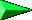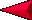NEXT
BACK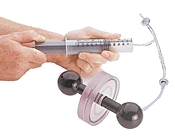?Mechanical Arm
- pneumatics